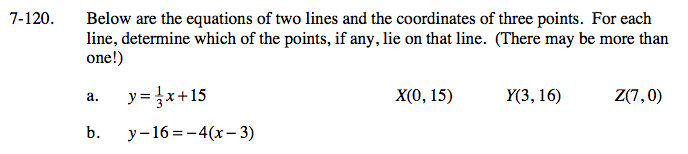### Home > GC > Chapter 7 > Lesson 7.3.3 > Problem7-120

7-120.Individually check to see if X, Y, and Z are on the line.
Do this by substituting the given coordinates into the original equation.
There may be more than one point correct!

$15 = \frac{1}{3}(0) + 15$

X is on the line.

$16 = \frac{1}{3}(3) + 15$

Y is on the line.

$0 \ne \frac{1}{3}(7) + 15$

Z is not on the line.

Follow the same procedure in part (a).